## 声学回声消除(Acoustic Echo Cancellation)

回声就是声音信号经过一系列反射之后，又听到了自己讲话的声音，这就是回声。一些回声是必要的，比如剧院里的音乐回声以及延迟时间较短的房间回声；而大多数回声会造成负面影响，比如在有线或者无线通信时重复听到自己讲话的声音（回想那些年我们开黑打游戏时，如果其中有个人开了外放，他的声音就会回荡来回荡去）。因此消除回声的负面影响对通信系统是十分必要的。

针对回声消除（Acoustic Echo Cancellation，AEC ）问题，现如今最流行的算法就是基于自适应滤波的回声消除算法。本文从回声信号的两种分类以及 AEC 的基本原理出发，介绍几种经典的 AEC 算法并对其性能进行阐释。

# 回声分类

在通信系统中，回声主要分为两类：电路回声声学回声

## 电路回声

电路回声通常产生于有线通话中，而造成电路回声的根本原因是转换混合器的二线-四线阻抗不能完全匹配。中心局至转换混合器之间采用四线的连接方式传输信号，上面两条线路用于发送给用户端信号，下面两条线路用于接收用户端信号。通信公司为了降低远距离信号传输成本，将混合器至用户端的连接线减少为二线连接，分别用于用户端信号的接受与发送。中间的转换混合电路功能是将四线连接转换为二线连接，由于在转换过程中使用了不同型号的电线或者负载线圈没有被使用的原因，不可避免地会产生阻抗不匹配现象，导致混合器接收线路上的语音信号流失到了发送线路，产生了回声信号，使得另一端的用户在接收信号的同时听到了自己的声音。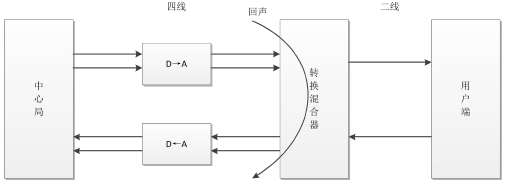在现如今的数字通信网络中，转换混合器与数模转换器融为一体，但无论是模拟电子线路还是数字电子线路，二-四线的转换都会造成阻抗不匹配问题，从而导致其产生电路回声，影响现代通信质量。由于电路回声的线性以及稳定性，用一个简单的线性叠加器就可以实现电路回声消除。首先将产生的回声信号在数值上取反，线性地叠加在回声信号上，将产生的回声信号抵消，实现电路回声的初步消除。然而由于技术缺陷，线性叠加器不能完整地将回声信号抹去，因此需要添加一个非线性处理器，其实质是一个阻挡信号的开关，将残余的回声信号经过非线性处理之后，就可以实现电路回声的消除，或者得到噪声很小的静音信号。由于电路回声信号是线性且稳定的，所以比较容易将其消除，而本文主要研究的是如何消除非线性的声学回声。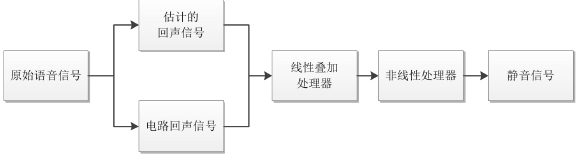## 声学回声

在麦克风与扬声器互相作用影响的双工通信系统中极易产生声学回声。如下图所示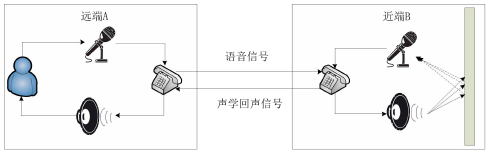直接回声不受环境的印象，与扬声器到麦克风的距离及位置有很大的关系，因此直接回声是一种线性信号

间接回声的大小与房间环境、物品摆放以及墙面吸引系数等等因素有关，因此间接回声是一种非线性信号

# AEC的基本原理

如今解决 AEC 问题最常用的方法，就是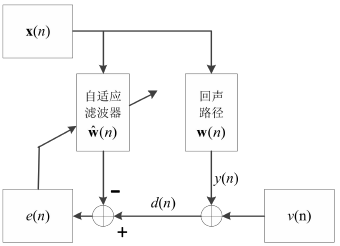AEC的基本原理

$x(n)$为远端输入信号，经过未知的回声路径$w(n)$得到$y(n)=x(n)*w(n)$，再加上观测噪声$v(n)$即为期望信号$d(n)= y(n) + v(n)$。x(n)通过自适应滤波器$\hat{w}(n)$得到估计的回声信号，并与期望信号$d(n)$相减得到误差信号$e(n)$，即$e(n)=d(n)-\hat{w}^T(n)x(n)$，误差信号的值越小说明自适应滤波算法所估计的回声路径就越接近实际的回声路径。

滤波器采用特定的自适应算法不停地调整权值向量，使估计的回声路径 \hat{w}(n) 逐渐趋近于真实回声路径$w(n)$。显然，在 AEC 问题中，自适应滤波器的选择对回声消除的性能好坏起着十分关键的作用。

# 自适应滤波器的基本原理

自适应滤波器是一个对输入信号进行处理并不停学习，直到其达到期望值的器件。自适应滤波器在输入信号非平稳条件下，也可以根据环境不断调节滤波器权值向量，使算法达到特定的收敛条件，从而实现自适应滤波过程。

自适应滤波器按输入信号类型可分为模拟滤波器和离散滤波器，本文中使用的是离散滤波器中的数字滤波器数字滤波器按结构可划分为输入不仅与过去和当前的输入有关、还与过去的输出有关的无限冲激响应滤波器（IIR），以及输出与有限个过去和当前的输入有关的有限冲激响应滤波器（FIR））为了使得自适应滤波器具有更强的稳定性，并且具有足够的滤波器系数可以用来调整以达到特定的收敛准则，一般选取横向的 FIR 滤波器进行来进行回声的消除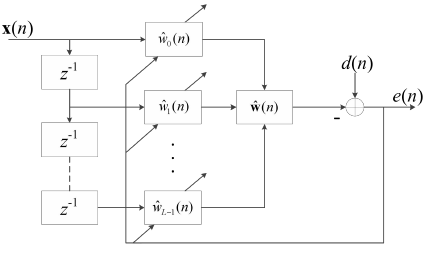横向FIR滤波器结构框图

$x(n)$是远端输入信号，$\hat{w}_i(n)$是滤波器系数，其中$i=0,1,...,L-1$，$L$为滤波器的长度，$n$为采样点数，$\hat{w}(n)$为滤波器的权值向量且$\hat{w}(n)=[\hat{w}_0(n),\hat{w}_1(n),...,\hat{w}_{L-1}(n)]^T$，根据误差信号$e(n)=d(n)-\hat{w}^T(n)x(n)$的值以及不同算法的收敛准则调整滤波器的权值向量。

然而自适应滤波算法的选择从根本上决定了回声消除的效果是否良好，接下来将介绍几种解决 AEC 问题的经典自适应滤波算法。

# 回声消除常用算法

## LSM算法

通过上面AEC的基本原理我们知道了误差信号$e(n)$等于期望信号减去滤波器输出信号：

$$e(n)=d(n)-\hat{w}^T(n)x(n)$$

$$\xi=E[e^2(n)]=E[d^2(n)]-2P^T\hat{w}(n)+\hat{w}^T(n)R\hat{w}(n)$$

对误差信号求导并且使导数值置零，求解得到使得误差最小的“最优权重” $\hat{w}_{opt}(n)=\frac{P}{R}$，R 和 P 的估计分别为$\hat{R}(n) 和$\hat{P}(n)$，利用各自的瞬时估计值将其分别表示为：\hat{R}(n)=x(x)x^T(n)；\hat{P}(n)=d(n)x(n) 。另外，用$\hat{g}_w(n)$表示误差信号对权值向量导数的估计值，利用下式方法求解最优权值向量的维纳解：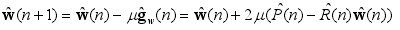得到：$\hat{g}_w(n)=-2e(n)x(n)$，算法取瞬时平方误差作为目标函数，那么$\hat{g}_w(n)$为其真实梯度，因为：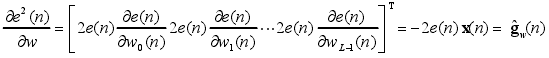因此得到 LMS 算法的权值向量更新公式： $$\hat{w}(n+1)=\hat{w}(n)+2\mu e(n)x(n)$$ 式中，$\mu$为固定步长因子，$\mu\$的大小很大程度上决定了算法的收敛与稳态性能。LMS 算法复杂性低，但是它的收敛速度慢。为改善 LMS 这个不足之处，科研人员提出一系列改进算法，NLMS 算法就是其中一种

# 参考

《基于自适应滤波器的声学回声消除研究——冯江浩》